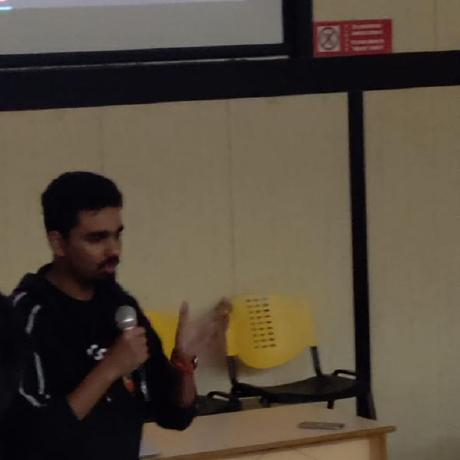×

Search anything:

# Different ways to terminate a program in Python

#### Python Software EngineeringIn this article, we are going to explore different ways in which we can terminate and exit a python program.

1. Using the quit function
2. Using the sys.exit method
3. Using the exit() function
4. Using the `os._exit(0)` function
5. Using KeyBoard Interrupt and raise systemexit

Let us get started with Different ways to terminate a program in Python.

## Using the quit function

Using the python quit function is a simple and effective way to exit a python program. No external imports are required for this.

``````print("Program Start")
print("Using quit()")
quit()
print("Program End")
``````

The output for this will be

``````python .\exit.py
Program Start
Using quit()
``````

The program ends after encountering the quit function and the last line is not printed.

## Using the sys.exit method

The python sys module can be imported and used . It is used to manipulate the python runtime and the exit method can be used to end the program.

``````import sys
print("Program Start")
print("Using sys.exit()")
sys.exit("System Exiting")
print("Program End")
``````

This program like the previous one will end after encountering the sys exit method.
Output of this program is

``````python .\exit.py
Program Start
Using sys.exit()
System Exiting
``````

## Using the exit() function

This is similar to the quit function. It is inbuilt in python

``````print("Program Start")
print("Using exit()")
exit()
print("Program End")
``````

The output of this is similar

``````python .\exit.py
Program Start
Using exit()
``````

## Using the os._exit(0) function

The os package comes with a method to exit the process with a specified status, it does not need to call cleanup handlers, flushing stdio buffers, etc.

Here in our example we are using it in a child process after calling os.fork

``````import os
pid = os.fork()
if pid > 0:
print("\nThis is the main parent process")
info = os.waitpid(pid, 0)
if os.WIFEXITED(info) :
exit_code = os.WEXITSTATUS(info)
print("Exit code of the child:",exit_code)
else:
print("This is child process")
print("PID of child process is :", os.getpid())
print("Child is now exiting")
os._exit(os.EX_OK)
``````

The program creates a child process and once it is done program terminates by using the os._exit() function.

``````This is the main parent process
This is child process
PID of child process is: 294
Child is now exiting
Exit code of the child: 0
``````

## Using KeyBoard Interrupt and raise systemexit

The KeyBoardInterrupt is an exception which is raised when user interrupts a running python script by hitting Ctrl+C or Ctrl+Z. If the Python program does not catch the exception, then it will cause python program to exit. If the exception is caught then it may prevent Python program to exit.

The systemexit is an exception which inherits from the python BaseException and can be raised to make a program exit.

``````import time
try:
count=0
while 1==1:
count=count+2
print(f"Value of count {count}")
time.sleep(1)
except KeyboardInterrupt:
print("Raising SystemExit")
raise SystemExit
``````

The program uses a try catch block to run a loop which updates a variable count value. When a KeyBoardInterrupt is caught it raises the SystemExit exception to terminate and exit the program.

The output of the program is

``````python .\exit.py
Value of count 2
Value of count 4
Value of count 6
Value of count 8
Value of count 10
Value of count 12
Raising SystemExit
``````

These are some of the different ways to terminate and end a python program. With this article at OpenGenus, you must have the complete idea of how to terminate / exit a given Python program.#### Dipto Chakrabarty

Third year student from VIT Vellore in Computer Science with specialization in Information Security.

Different ways to terminate a program in Python